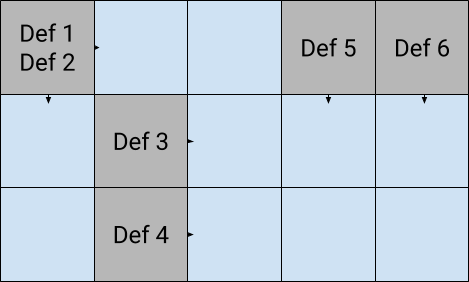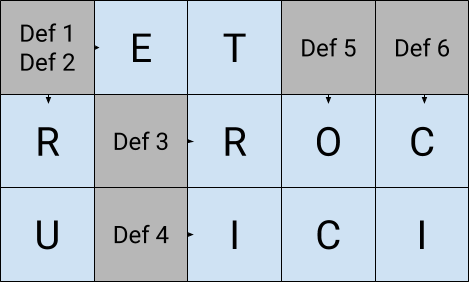# Use SMT Solvers to generate crossword grids (2)

#### development z3

This post is part of a series on using SMT Solvers to generate crossword grids.

Thanks @geistindersh for his feedback, and corrections!

In a previous blog post we presented SMT Solvers, and mentioned that we can use them to solve problems; More explicitly, for our problem at hand, we plan to:

• Construct a formula to represent (or encode) “generic” crossword grids;
• Ask the Solver to give us a solution (a set of values);
• Interpret this solution (or decode) to obtain a valid, completed, crossword grid.

$\text{grid} \xrightarrow[]{\text{encode}} \text{formula} \xrightarrow[]{\text{solve}} \text{values} \xrightarrow[]{\text{decode}} \text{completed grid} \\$

In this post, we will first define some vocabulary and definitions we use while constructing our solution. Then we are going to state more clearly what we are aiming to achieve. At the end, we will present some formulas, the building blocks of how we can represent any crossword grid.

# Crosswords

## Definitions

Let’s clarify some concept that we are going to use to describe crossword grids:

• A grid is composed of: definition slots, and (empty) cells;
• Definitions do not take more room than a cell, and there can be up to two definitions per slot;
• Following the definitions, words can be written vertically (from top to bottom), and horizontally (from left to right).

Here is a basic example of what an empty crossword grid could look like:The aim of a game of crosswords is to fill a grid with words taken from the dictionary, (preferably) respecting the definitions, with each cell containing one (and only one) letter.

Here is a valid solution of the previous example, using French words (by chauvinism):## So, back to the problem: What are we trying to do?

Let’s not care about definitions. If we can generate a grid of intersecting words respecting the rules:

• One and only one letter per cell;
• Words are coming from a list of valid words;
• Words are written left to right or top to bottom;

Then adding definitions is relatively straightforward, using a mapping of words to definitions (a.k.a., a dictionary).

# From grid to formula back to grid

Now that we specified what we talk about, let’s write some formulas; Gradually, from simple to complex.

As we mentioned earlier, we will use Z3, because it supports a String theory 1. Furthermore, we will make use of the Python bindings for Z3, to make the code friendly to beginners’ eyes.

## A single valid word

Let’s start with the simplest case we can think of: a grid composed of a single word. It would look like this:

Essentially, we declare a variable named $$horizontal$$ and constrain it to take any value from a finite wordlist (our dictionary).

# Using a Python REPL
python> horizontal = String('horizontal')

python> formula = Or(
horizontal == StringVal("abat"),
horizontal == StringVal("abbe"),
horizontal == StringVal("abri"),
# [...]
)


In the above example, we see that $$horizontal$$ can take the value abat, or the value abbe, or the value abri, etc. As you might guess, we truncated the display: dictionaries are get pretty big, so the formula is effectively thousands of lines long.

Note that if we consider $$horizontal$$ to be potentially any word from the dictionary, we would end with a massive formula, involving ten of thousands of disjunctions. The French wordlist we will use counts 200 224 entries! And that would only get worse for a complete grid, counting between 50 and 100 words (ballpark estimate)… Intuitively, we want to keep the “size of the queries” we send to the Solver relatively small for them to be able to handle the task.

There is something that we already know about $$horizontal$$ that we do not need the help of a Solver for: its size! Indeed, we know from the grid that $$horizontal$$ should be of length 4, hence, we don’t need to pass the Solver anything that involves words of size different than that.

In practice, instead of having a single wordlist, we use many: each one of them referencing only words of the same size. At most, that means we have 36 wordlists for the French language 2.

python> solver = Solver()
python> solver.check()
sat
python> solver.model()
[horizontal = "abbe"]


Which we can map back into the grid frame we had:

Yay! We generated a first single word grid!

## Two valid words

Let’s up the game, because crossword grids would either get boring or very frustrating with a single word to guess. Consider the following:

In the same spirit than earlier: we now use $$horizontal$$ and $$vertical$$, two variables that can take any values from respectively two different wordlists, the first being the list of French words of length 4, the latter the list of French words of length 2 (again, we omit possible values for brevity):

python> horizontal = String('horizontal')
python> vertical = String('vertical')

python> formula = And(
Or(
horizontal == StringVal("abat"),
horizontal == StringVal("abbe"),
horizontal == StringVal("abri"),
# [...]
),
Or(
vertical == StringVal("ah"),
vertical == StringVal("an"),
vertical == StringVal("ru"),
# [...]
)
)

python> solver = Solver()
python> solver.check()
sat
python> solver.model()
[vertical = "ru", horizontal = "abbe"]


Again, the Solver was able to find a solution, that we interpret as the following filled grid:

## Two valid intersecting words

Disconnected words do not represent very well the content of real world crossword grids. Next step is to start assembling:

From the formula perspective, we start exactly as earlier, and then we add a constraint saying that the character at index $$2$$ of $$horizontal$$ must equal the character at index $$0$$ of $$vertical$$.

python> horizontal = String('horizontal')
python> vertical = String('vertical')

python> formula = And(
Or(
horizontal == StringVal("abat"),
horizontal == StringVal("abbe"),
horizontal == StringVal("abri"),
# [...]
),
Or(
vertical == StringVal("ah"),
vertical == StringVal("an"),
vertical == StringVal("ru"),
# [...]
),
horizontal == vertical
)

python> solver = Solver()
python> solver.check()
sat
python> solver.model()
[vertical = "ru", horizontal = "abri"]


And the given solution is translated to the following grid:

Note that the result is (as in the previous examples) one of many valid combinations: there are other four letter words where the character at index $$2$$ is the same as the character at index $$0$$ of a well chosen two letter word.

Boom, we now have everything we need to represent complete crossword grids!

In the next (and last) blog post, we will present the complete formula we build, and the results we obtain trying to generate grids this way.

1. Under this theory, variables take their values among all the possible sequences of characters, and operations can be substring comparison, concatenation, etc.

2. According to the relevant Wikipedia page, the longest French word is “hippopotomonstrosesquippedaliophobie”, counting 36 letters!